# 「BUAA OO」第一单元总结

## 零、任务简介

• 第一次作业只有常数、单独的自变量x、单层括号，实现的是：$$(x+1)*(x+2)$$ -> $$x**2+3*x+2$$

• 第二次作业引入了三角函数、自定义函数、求和函数，实现的是：$$x*(sum(i,1,10,(sin(x)+i)))$$ -> $$10*x*sin(x)+55*x$$

• 第三次作业引入了表达式因子，实现的是：$$(x+1*sin((x+2*(x+3)))$$ -> $$sin((3*x+6))+x$$

## 二、核心架构

#### 堆栈解析部分

1. 先进行一些预处理，如去掉空格、把 -++ 换为 -、把 ** 换为 ^

2. 然后根据运算符的优先级将中缀表达式转换成后缀表达式：

(x+1)^2 -> x 1 + 2 ^(3*sin(x))^2 -> 3 sin(x) * 2 ^

(f(x,sin(x))+1)*sum(i,1,2,x) -> f(x,sin(x))+1) 1 + sum(i,1,2,x) *

3. 再依序取出运算符、“数”（实际上需要先实例化为一个支持运算的对象，具体见”数据结构定义“），直接进行运算

4. 最后输出栈中唯一所剩的“数”即可

#### 数据结构定义

$\sum_{i=0}^8k_i*x^i$

public Factor add(Factor second) {
BigInteger[] result = new BigInteger;
for (int i = 0; i < result.length; i++) {
}
return new Factor(result);
}

public Factor mul(Factor second) {
BigInteger[] result = new BigInteger;
Arrays.fill(result, BigInteger.valueOf(0));
for (int i = 0; i < result.length; i++) {
for (int j = 0; j <= i; j++) {
result[i] = result[i].add(factors[j].multiply(second.getFactors(i - j)));
}
}
return new Factor(result);
}


$\sum_{i}(k_i*x^{a_i}\times\prod_{j}sin^{b_{ij}}(p_{ij}*x^{m_{ij}})\times\prod_{l}cos^{c_{il}}(q_{il}*x^{n_{il}}))$

$$Expr: ArrayList$$< $$Factor$$ >$$\ factors$$

$$Factor:BigInteger\ k、BigInteger\ a、ArrayList$$< $$Sin$$ >$$\ sins、ArrayList$$< $$Cos$$ >$$\ coses$$

$$Sin:BigInteger\ b、BigInteger\ p、BigInteger\ m$$

$$Cos:BigInteger\ c、BigInteger\ q、BigInteger\ n$$

• Factor 间的乘法：

public Factor mul(Factor factor) {
BigInteger k = this.k.multiply(factor.getK());
BigInteger a = this.a.add(factor.getA());
ArrayList<Sin> sins = new ArrayList<>(this.sins);
ArrayList<Cos> coses = new ArrayList<>(this.coses);
return new Factor(k, a, sins, coses);
}

• Expr 间的运算（以加法与乘法为例）：

public Expr add(Expr expr) {
ArrayList<Factor> factors = new ArrayList<>(expr.getFactors());
return new Expr(factors);
}

public Expr mul(Expr expr) {
ArrayList<Factor> factors = new ArrayList<>();
for (Factor factor : this.factors) {
for (int j = 0; j < expr.getFactors().size(); j++) {
}
}
return new Expr(factors);
}


$\sum_{i}(k_i*x^{a_i}\times\prod_{j}sin^{b_{ij}}(Expr_{ij})\times\prod_{l}cos^{c_{il}}(Expr_{il}))$

$$Sin:BigInteger\ b、Expr\ expr$$

$$Cos:BigInteger\ c、Expr\ expr$$

#### 小结

（很有意思的一点是我面向过程的函数在三次作业中基本不用修改，都是很核心的代码，每一次作业的迭代仅仅是需要在同一内核的基础上向上不断加强扩展封装；反而是对象相关的部分，由于数据结构特化的原因，每次都要重构不少、扩展性明显较弱）

## 三、具体结构与度量分析

### 第一次作业

#### 1、结构示意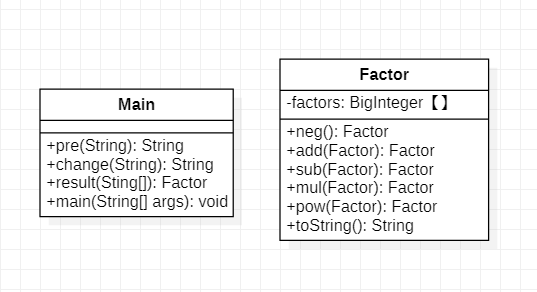pre：一些预处理

change：中缀表达式 -> 后缀表达式

result：后缀表达式 -> 最终的 Factor 结果

#### 2、度量分析

1）方法复杂度分析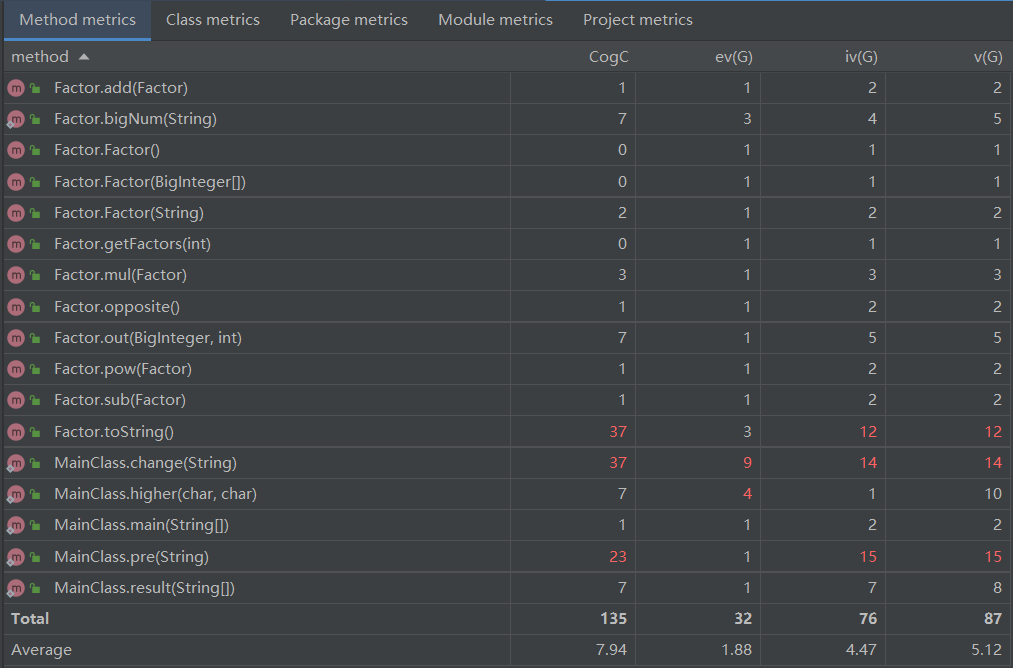2）类复杂度分析### 第二次作业

#### 1、结构示意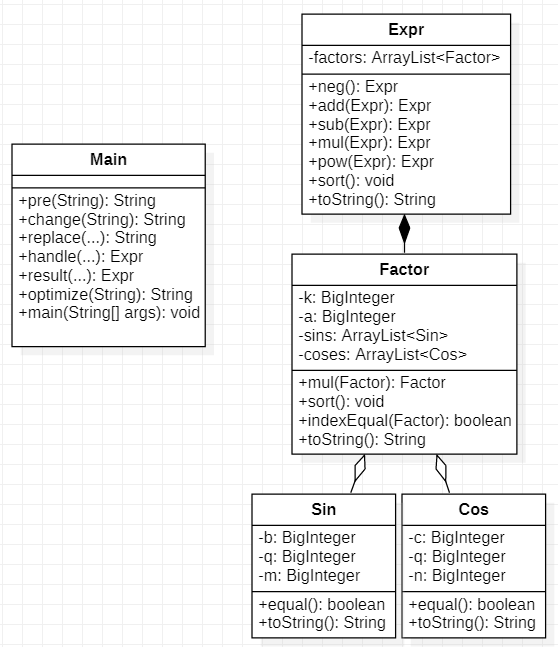replace：进行自定义/求和函数的替换

handle：进行自定义/求和函数的处理计算

optimize：优化模块

sort：整理模块（其实应该是sort out/arrangement...为了方便就直接命名为sort了）

#### 2、度量分析

1）方法复杂度分析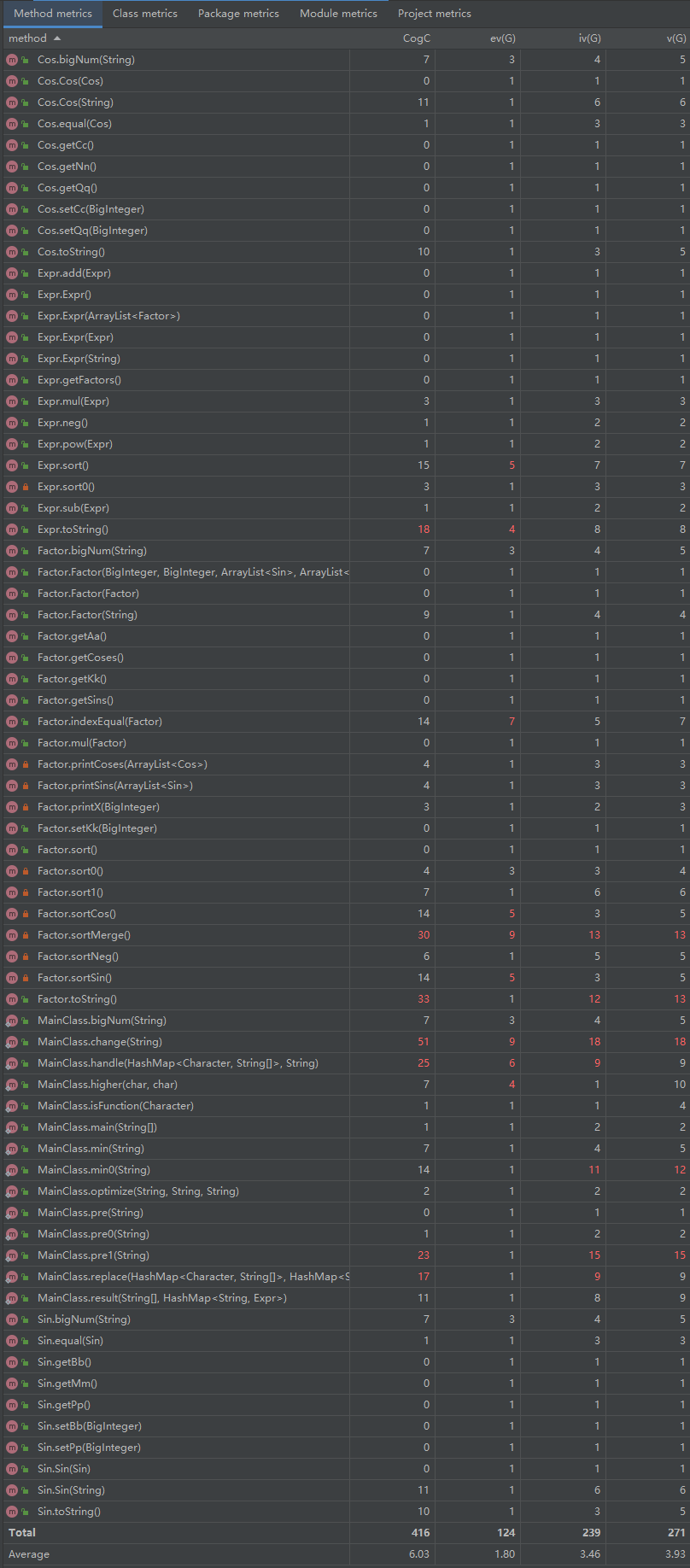2）类复杂度分析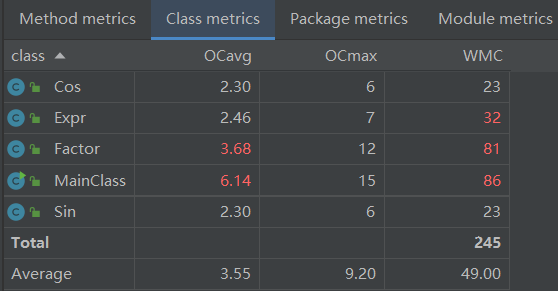### 第三次作业

#### 1、结构示意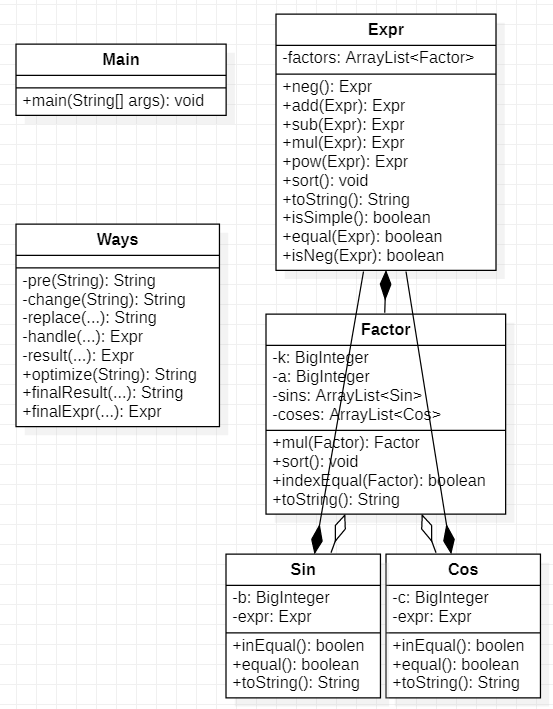finalResult = pre+change+replace+handle+result+optimize（对函数进行了封装，便于实现递归）

finalExpr = finalResult+pre+change+replace+handle+result（产生 Expr 以供递归使用）

isSimple：判断一个表达式是否是一个简单表达式，即能否直接作为一个简单因子（三角函数内是简单因子则不用再加括号）

#### 2、度量分析

1）方法复杂度分析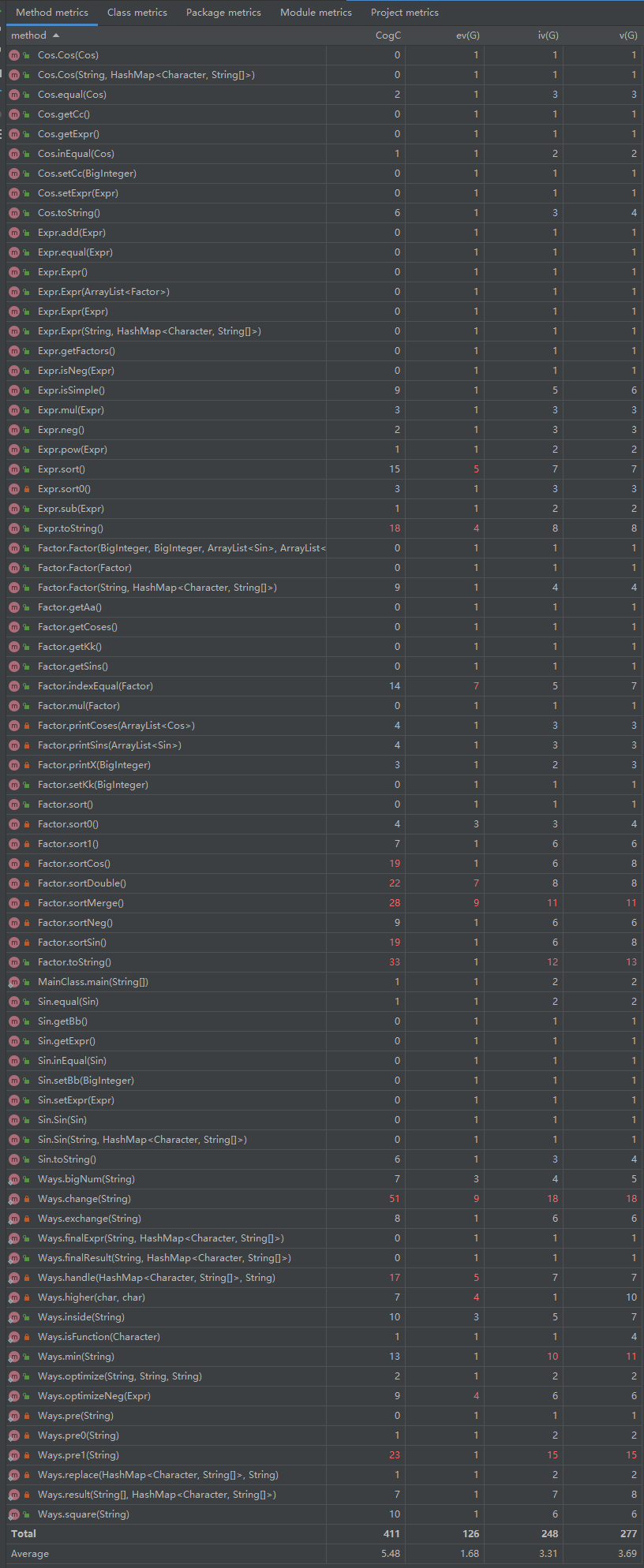2）类复杂度分析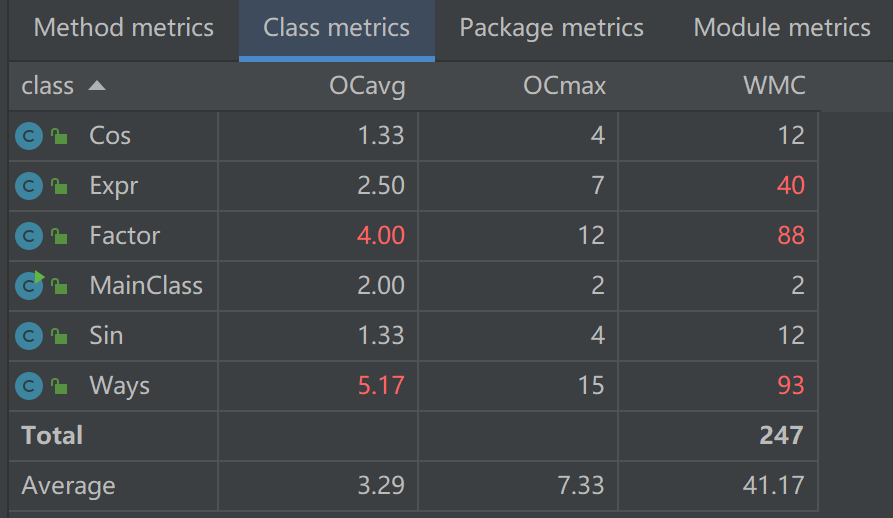## 四、优化相关

### 第一次作业

1. 正项的提前：如 -x+1 -> 1-x
2. 平方项的优化：x**2 -> x*x

### 第二次作业

#### Factor 层次

• sort：自整理，包含以下部分：

• sort0：搜寻系数k、sin内是否为0，为0则整个 Factor 直接为0
• sort1：搜寻sin、cos的指数是否为0，为0则可以从 ArrayList 里去掉该sin/cos
• sortNeg：搜索sin、cos内的式子是否为负，为负则提出负号
• sortMerge：合并括号内的式子相等的sin/cos
• sortSin：给 ArrayList<sin> 实现一个内部排序（便于后续比对）
• sortCos：给 ArrayList<cos> 实现一个内部排序（便于后续比对）

#### Expr 层次

• sort：自整理，包含以下部分：
• 对每个 factor 自整理一次
• sort0：搜索是否有首系数为0的 factor，为0则可以从 ArrayList 里去掉该 factor
• 合并：合并除系数以外各项参数相同的 factor

#### 顶层

• optimize：进行三角函数的转换优化（打表式暴力优化）
• $$sin^2(x)$$ 直接替换为 $$(1-cos^2(x))$$
• 调用 finalResult 函数，把再跑一次的结果存下来
• 再进行诸如 $$cos^2(x**2)$$ -> $$(1-sin^2(x**2))$$$$sin^2(1)$$ -> $$(cos^2(1)-cos(2))$$ 的替换，重复上述2步
• 将存取的多个表达式进行比较，挑取最短的输出

### 第三次作业

#### 平方优化

1. 满血版：暴力搜索所有三角函数平方项，均进行替换、再计算并存取
2. 残血版：只进行部分特判，如$$sin^2(x)$$$$sin^2(x**2)$$ ，不考虑 $$sin^2((x+1))$$ 等复杂情况

## 五、测试与debug

### 测试

#### 自测

1. 代码的功能性分割强，主要做的是局部功能测试
2. 设计架构清晰，自己相对比较清楚容易出 bug 的地方，故针对性搓数据即可
3. 时间有限（高工这学期的课有一点点多，我一般是周五凌晨才开始写OO，每次作业的平均总用时在12小时左右，特别是第一次作业是在8小时内赶完的......第一次没空写测试程序导致我后面也懒得写了
4. 自己对优化板块更感兴趣，动力比较足，所以重优化轻测试了

### debug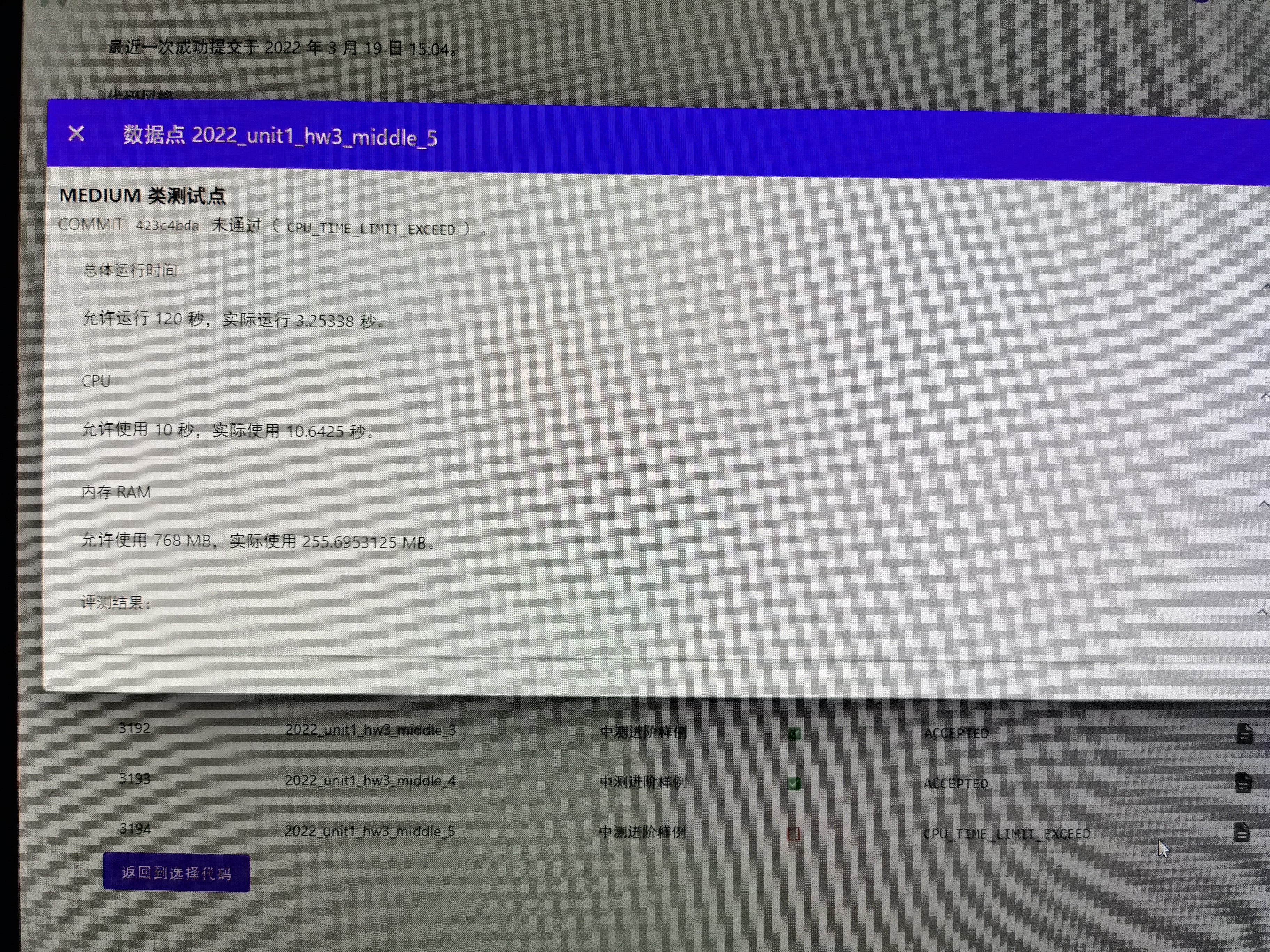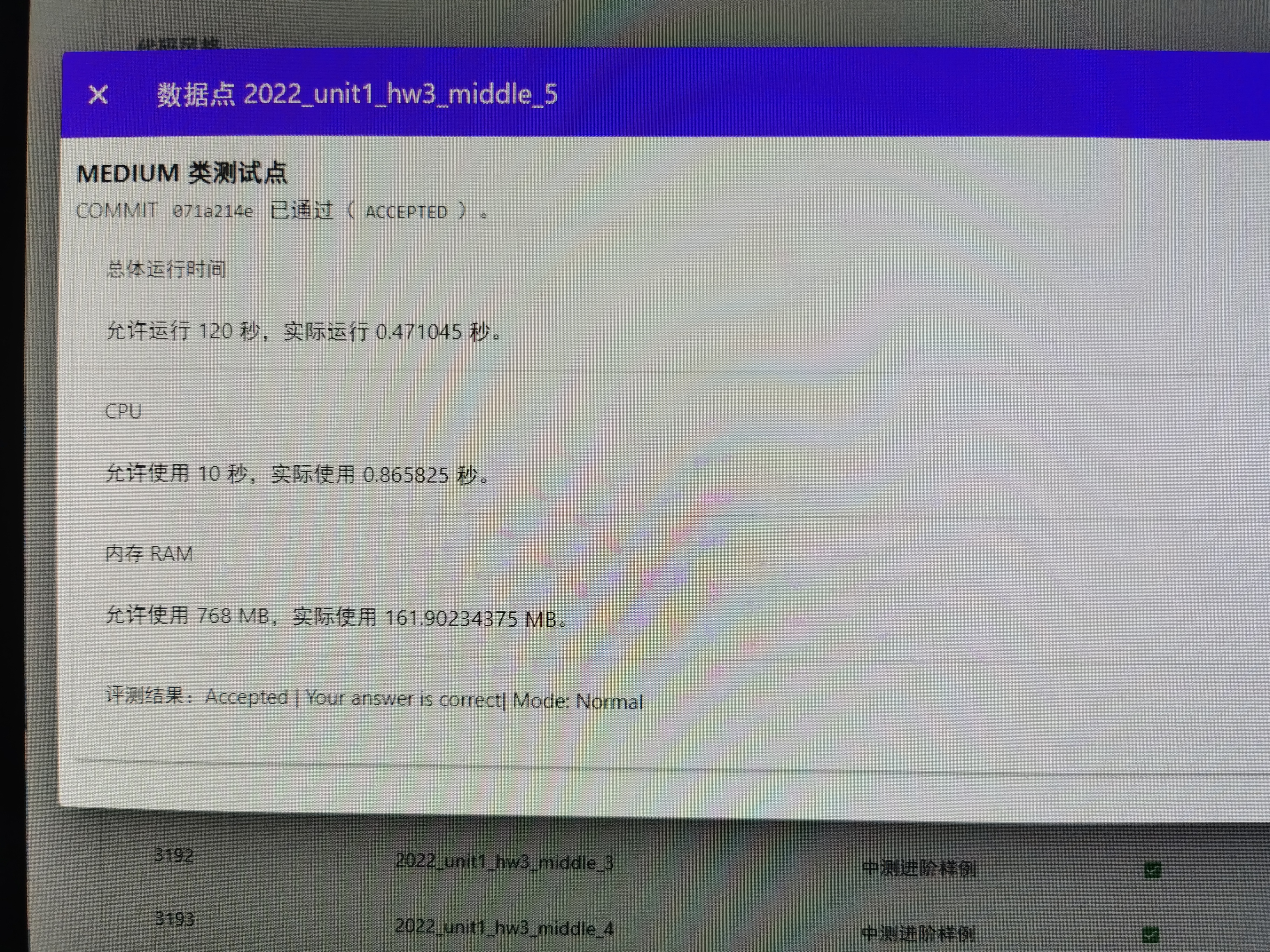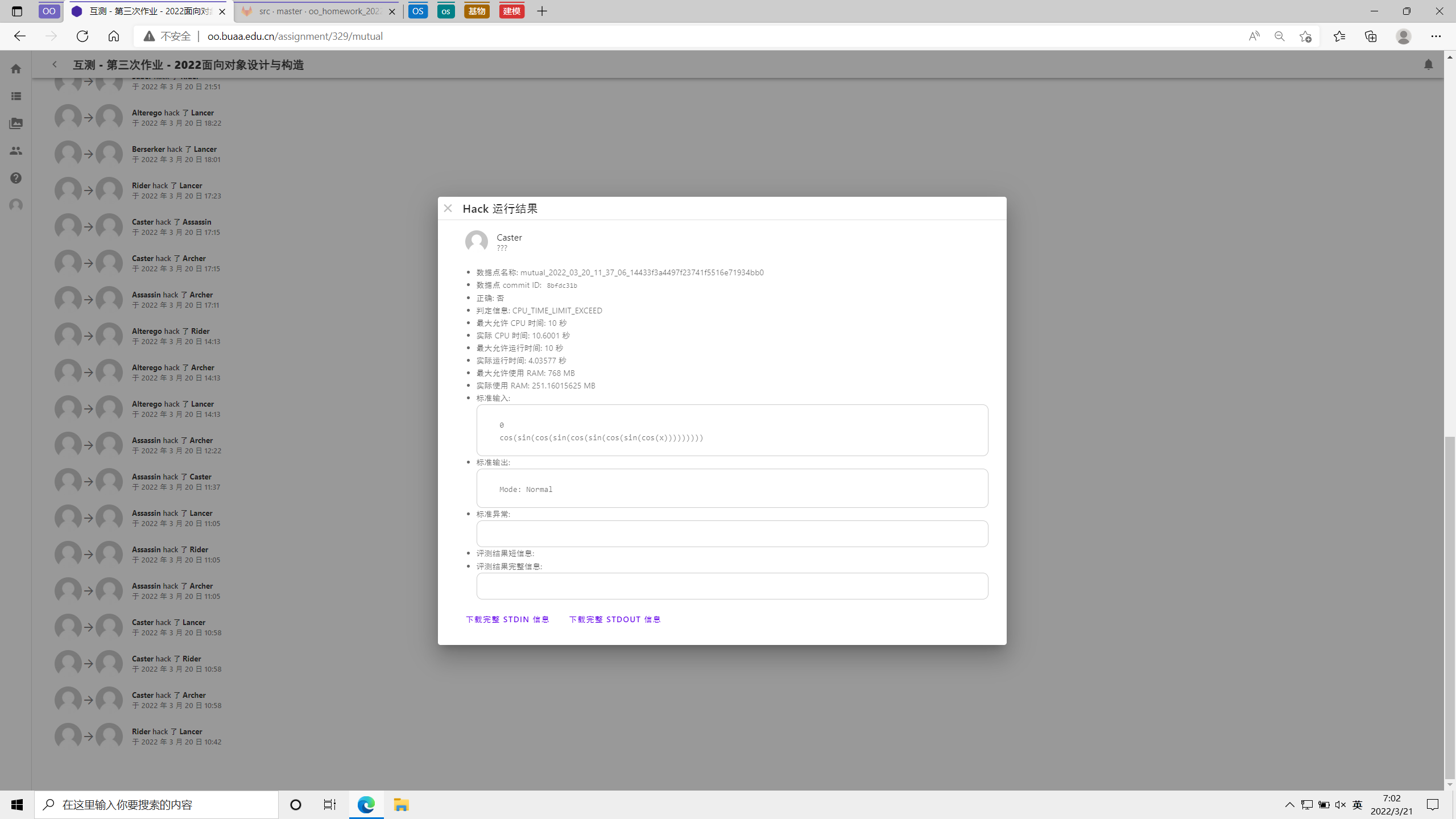bug 修复很简单，进一步注释掉剩下的优化模块即可，这样程序运行起来就快得多了（）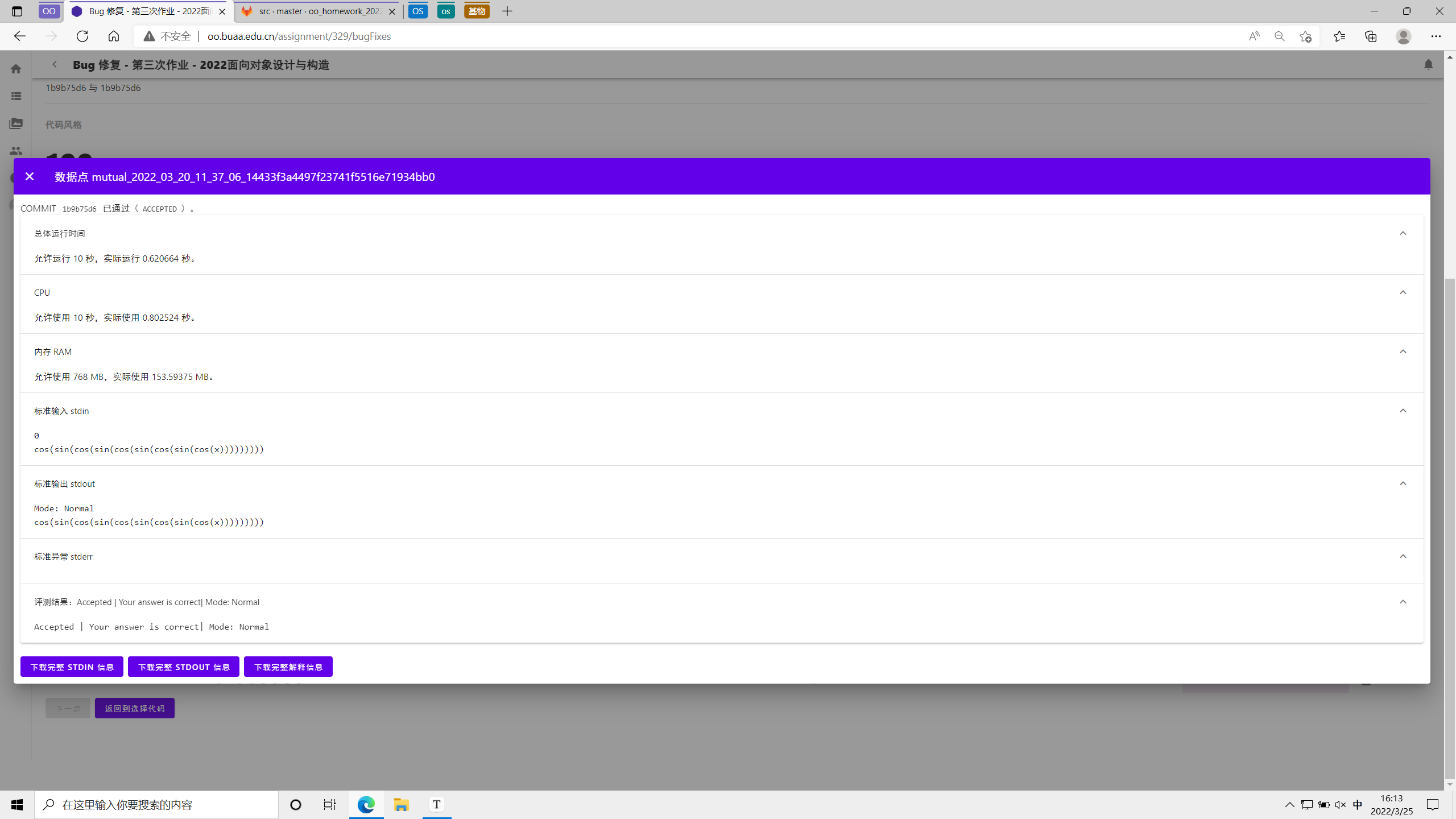## 六、心得体会

### 面向对象 or 面向过程？

1. 字符串这一待解析形式。

可以做一个比较：pre2 中的冒险者游戏就是一个典型的很适合面向对象的形式主题，个体自身的整体性明显，操作都是一条一条、及其清晰准确的，于是在教程的引导下我们也都建立了合适的抽象、继承等逻辑，初步体会到了面向对象的设计思想；

而对于字符串处理，要解析的话，怎么着也得先读入、接着拿去建类、建好了类再拿回来建立层次结构（其中可能还涉及到所谓”递归下降“的方法）......官方 training 里的 Parser、Lexer 大概就是这样分步做了解析——总之是无法从一开始就面向对象的。

我认为在思考自己的方法“是否面向对象”的时候，解析板块，也即本单元的内核，绝对是不容忽略的。

2. 对于”表达式树“的本质理解。

在大量阅读其他同学的博客后（毕竟我这儿交得比较晚嘛），我发现大家对于“表达式树”各有理解：有的同学主要阐述了递归下降法并给出了图例，有的同学甚至直接明确指出“本单元不需建立表达式树”，还有的同学“在转换为后缀表达式后再建立了表达式树”......

我的观点与一部分同学的观点一致——无论自己有没有意识到，本单元的解析处理过程本质上全是在“建立表达式树”的过程（递归下降就是在延展树的深度；而栈堆与树本质上本来就是等价的）。

在我看来，”层次化“的过程本身无关面向对象/面向过程，关键还是层次化的具体内容。pre2 里冒险者、装备的层次化无疑就很面向对象；而本单元里的表达式树我认为其本质上就很面向过程。

1. 本单元作业的设计本身就不是很纯粹的面向对象。

2. 面向过程/面向对象归根结底都只是设计思想罢了，终究还是为实现设计目标而服务的。当然，在《面向对象设计与构造》这门课程中我们自然更应该去尝试培养自己面向对象的设计思想；但我认为具体情况还是可以具体分析的，即使在OO这门课程里，我觉得也没必要对面向过程讳莫如深，清晰合适的架构可能更为重要。

（最后这一部分主观感受居多，恳请大家批评指正、欢迎讨论交流！）

posted @ 2022-03-26 02:05  郑星宇  阅读(99)  评论(0编辑  收藏  举报